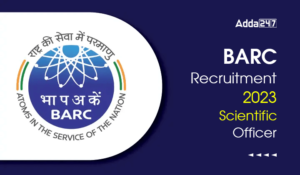Engineering Jobs   »   Mechincal Engineering quizs   »   NHPC JE Recruitment 2021, NHPC JE...

# NHPC JE’21 ME: Daily Practices Quiz. 26-Nov-2021

Quiz: Mechanical Engineering
Exam: NHPC JE
Topic: Thermodynamics

Each question carries 1 mark
Negative marking: 1/4 mark
Time: 6 Minutes

Q1. For a simple closed system of constant composition, the difference between the net heat and work interactions is identifiable as the change in
(a) Enthalpy
(b) Entropy
(c) Flow energy
(d) Internal energy

Q2. A closed system undergoes a process 1-2 for which the values of Q_(1-2) and W_(1-2) are + 20kJ and + 50kJ, respectively. If the system is returned to state 1 and Q_(2-1) is –10kJ, what is the value of the work W_(2-1) ?
(a) +20 kJ
(b) -40 kJ
(c) -80 kJ
(d) +40 kJ

Q3. Consider the following statements:
A real gas obeys perfect gas law at very
1. high temperatures
2. high pressures
3. low pressures
Which of these statements is/are correct?
(a) 1 only
(b) 1 and 3
(c) 2 only
(d) 3 only

Q4. At critical point the enthalpy of vaporization is
(a) dependent on temperature only
(b) maximum
(c) minimum
(d) zero

Q5. An engine working on Carnot cycle rejects 40% of absorbed heat from the source, while the sink temperature is maintained at 27°c, then the source temperature is
(a) 750°C
(b) 477°C
(c) 203°C
(d) 67.5°C

Q6. The irreversibility is defined as the difference of the maximum useful work and actual work : I=W_max⁡useful -W_actual. How can this be alternatively expressed?
(a) I=T_0 (∆S_system+∆S_surrounding)
(b) I=T_0 (∆S_System-∆S_surrounding)
(c) I=T_0 (√(∆S_system )+√(∆S_surrounding ))
(d) I=T_0 (√(∆S_system )-√(∆S_Surroundings ))

Solutions

S1. Ans.(d)
Sol. The given statement is for first law of Thermodynamics i.e.
δQ=dU+δW
δQ-δW=dU

S2. Ans.(b)
Sol. For process 1 -2
U_1+Q=U_2+W
U_1-U_2=W-Q=50-20=30 kJ
For process 2 – 1
U_2+Q=U_1+W
Q+U_2-U_1=W
-10-30=W
W=-40 kJ

S3. Ans.(b)
Sol. A real gas starts behaving as ideal gas at low pressure and high temperature.

S4. Ans.(d)
Sol. At critical point enthalpy of vaporization is zero.

S5. Ans.(b)
Sol. Sink temperature: T_2=27°C = 27 + 273 = 300K
It is given that engine rejects 40% of absorbed heat from the source
For a Carnot cycle engine

Q_1/T_1 =Q_2/T_2
Q_1/T_1 =(0.4 Q_1)/300
⇒T=300/0.4=750 K
= 477°C

S6. Ans.(a)
Sol.
Irreversibility,
I=T_0 (∆S)_universe
=T_0 (∆S_system+∆S_surrounding)

Sharing is caring!

•GATE 2023 Admit Card Out, Download Link ...
•TSSPDCL Recruitment 2023 Notification Ou...
•ISRO IPRC Recruitment 2023 Notification ...
•SECL Recruitment 2023 Notification Out, ...
•BARC Recruitment 2023 Scientific Officer...
•Central Railway Recruitment 2023 Apply O...
•BSF Recruitment 2023, Apply Online for J...